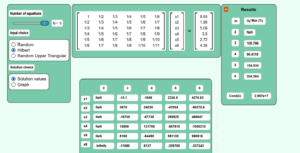# Chapter 04 Round-Off Error in Solution of Simultaneous Linear Equations Simulation

Description:  This simulation shows you the effect of round-off error in the solution of simultaneous linear equations.Keywords:  simultaneous linear equations, round-off error, significant digits.

Learning Objectives:  After successful completion of running the simulation with the student would be able to 1) find the effect of round-off errors in simultaneous linear equations using Naive Gauss elimination method.

Full Resources:  The full resources for the topic of the floating-point binary representation are given here which include textbook content, a PowerPoint presentation, a multiple-choice test, audiovisual lectures, and application examples.